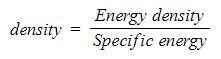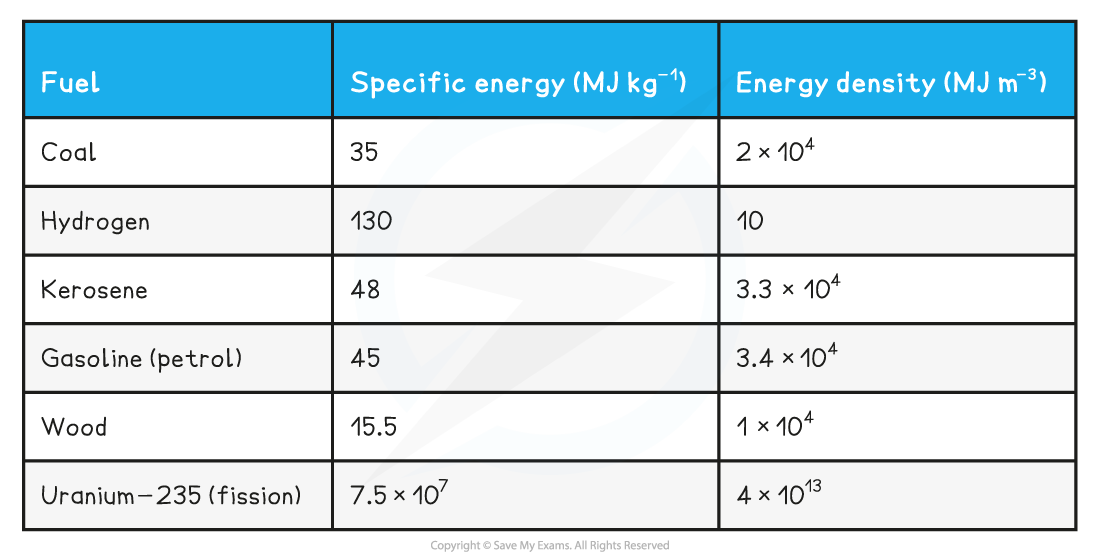# IB DP Physics: HL复习笔记8.1.1 Specific Energy

### Specific Energy

• The amount of energy that a fuel can provide for the amount of fuel used is an important consideration for the modern world
• In order to compare fuels by unit, either mass or volume can be used
• Comparing by mass is known as specific energy
• Comparing by volume is known as energy density
• Specific energy (ES) is a measure of the amount of energy per unit mass of a fuel
• It is measured in J kg-1
• Energy density (ED) is a measure of the amount of energy per unit volume of a fuel
• It is measured in J m-3
• The formula used to calculate density from specific energy and energy density is:
•Energy density is related to specific energy by the density of relevant fuel and can be converted between the two quantities this value of density

#### Worked Example

Use the values of specific energy for coal: 35 MJ kg-1 and energy density for coal: 2 × 104 MJ m-3

Estimate the approximate density for coal.

Step 1: List known values

• Specific energy: 35 MJ kg-1
• energy density: 2 × 104 MJ m-3

Step 2: Identify relationship needed

• Density has units of kg m-3
• Therefore, energy density must be divided by specific energy

Step 3: Perform the division

ED ÷ SE = (2 × 104) ÷ 35 ≈ 571 kg m-3

Step 4: State the final answer

• The approximate density of coal is: 571 kg m-3

Examples of Common Fuels: Energy Density and Specific Energy Table#### Exam Tip

The term specific in physics means per unit mass. For example, in specific heat capacity, the unit is per unit mass (and per degree temperature). Make sure not to confuse specific energy and specific heat capacity which are different concepts though related as they both refer to mass and energy!